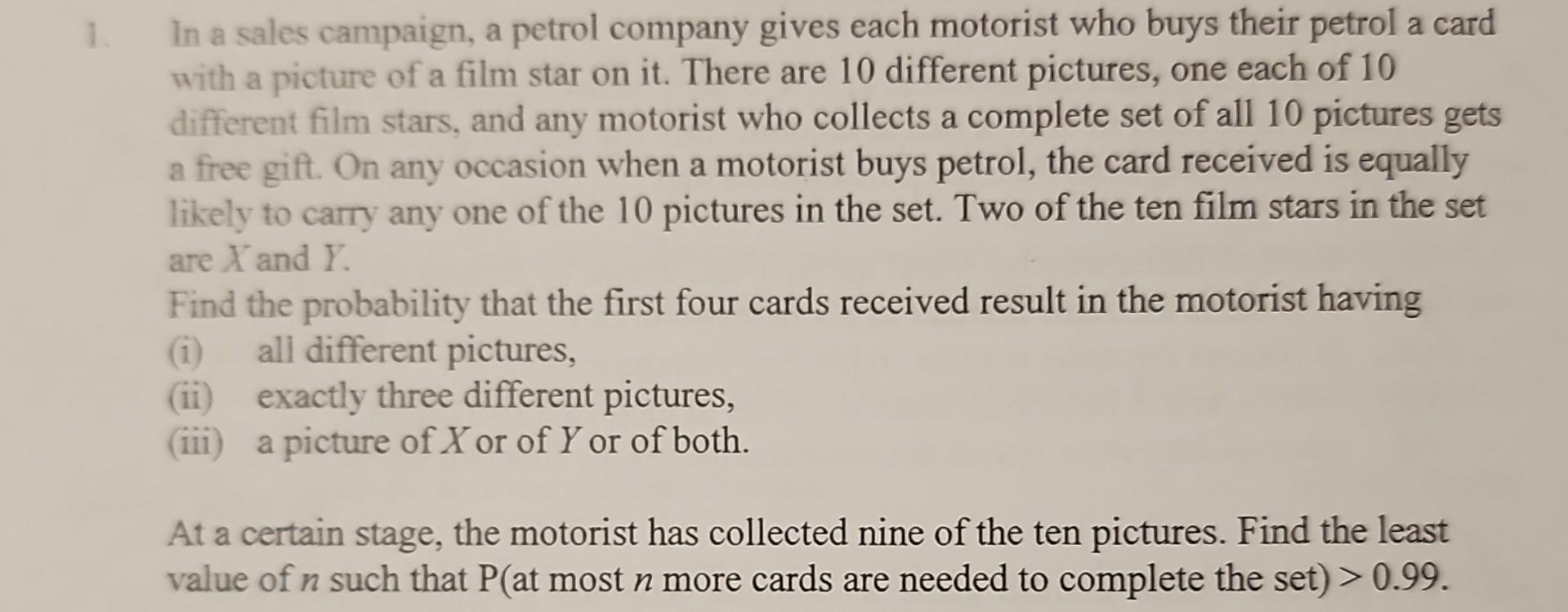# Question   Probability. Need step solution. Thanks in advance. In a sales campaign, a petrol company gives each motorist who buys their petrol a card with a picture of a film star on it. There are 10 different pictures, one each of 10 different film stars, and any motorist who collects a complete set of all 10 pictures gets a free gift. On any occasion when a motorist buys petrol, the card received is equally likely to carry any one of the 10 pictures in the set. Two of the ten film stars in the set are $$X$$ and $$Y$$. Find the probability that the first four cards received result in the motorist having (i) all different pictures, (ii) exactly three different pictures, (iii) a picture of $$X$$ or of $$Y$$ or of both. At a certain stage, the motorist has collected nine of the ten pictures. Find the least value of $$n$$ such that $$\mathrm{P}$$ (at most $$n$$ more cards are needed to complete the set $$)>0.99$$.Probability. Need step solution. Thanks in advance.

Transcribed Image Text: In a sales campaign, a petrol company gives each motorist who buys their petrol a card with a picture of a film star on it. There are 10 different pictures, one each of 10 different film stars, and any motorist who collects a complete set of all 10 pictures gets a free gift. On any occasion when a motorist buys petrol, the card received is equally likely to carry any one of the 10 pictures in the set. Two of the ten film stars in the set are $$X$$ and $$Y$$. Find the probability that the first four cards received result in the motorist having (i) all different pictures, (ii) exactly three different pictures, (iii) a picture of $$X$$ or of $$Y$$ or of both. At a certain stage, the motorist has collected nine of the ten pictures. Find the least value of $$n$$ such that $$\mathrm{P}$$ (at most $$n$$ more cards are needed to complete the set $$)>0.99$$.
More
Transcribed Image Text: In a sales campaign, a petrol company gives each motorist who buys their petrol a card with a picture of a film star on it. There are 10 different pictures, one each of 10 different film stars, and any motorist who collects a complete set of all 10 pictures gets a free gift. On any occasion when a motorist buys petrol, the card received is equally likely to carry any one of the 10 pictures in the set. Two of the ten film stars in the set are $$X$$ and $$Y$$. Find the probability that the first four cards received result in the motorist having (i) all different pictures, (ii) exactly three different pictures, (iii) a picture of $$X$$ or of $$Y$$ or of both. At a certain stage, the motorist has collected nine of the ten pictures. Find the least value of $$n$$ such that $$\mathrm{P}$$ (at most $$n$$ more cards are needed to complete the set $$)>0.99$$.